Electromagnetism

The branch of physics whose object is the electromagnetic field (i.e. the combination of electric and magnetic fields, which originally were two separate fields of study in the framework of statics, that is, without rapid time variations), which arises from a set of physical laws known as the Maxwell equations. Electromagnetism treats in an integrated fashion four basic areas in electricity and magnetism:

i) electrostatics, studying the forces acting between electric charges (a field studied by C.A. Coulomb, S.D. Poisson, C.F. Gauss, and others);

ii) magnetostatics, studying the forces among magnetic multipoles and magnetic materials (a field studied by Coulomb, Poisson, Gauss, J. Joule, and others),

iii) electromagnetism per se, studying the magnetic effects of electric currents (i.e. flowing electric charges), an effect discovered by H.C. Oersted and whose theoretical formulation owes much to A.M. Ampère;, and

iv) electromagnetic induction, studying the electrical effects of changing magnetic fields or situations (effects discovered by M. Faraday). Consequently, what is now (1998) known as Maxwell's equations (after J.C. Maxwell) and here presented following O. Heaviside and H.A. Lorentz, is the following set of first-order partial differential equations at any regular point(whether occupied by matter or in a vacuum; the electromagnetic field permeates all bodies to a greater or lesser extent) in Euclidean physical space:

Absence of magnetic monopoles: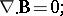(a1)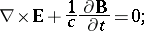(a2)

the Gauss–Poisson equation: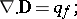(a3)

the Ampère equation: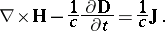(a4)

Here, the standard nabla formalism from vector analysis is used and all fields are functions of space () and time (). In these equations, which are expressed in a so-called laboratory frame and in Lorentz–Heaviside units (no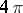and neithernor),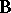andare the magnetic and electric induction (or displacement), whileandare the magnetic and electric fields;is the electric-current vector andis the volume density of free electric charges. The scalaris none other than the velocity of light in vacuum, a universal constant related to the ratio of electrostatic and magnetostatic units. The fields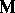anddefined by(a5)

are called electric and magnetic polarizations; the latter is simply called magnetization. Vacuum is defined by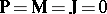and. These quantities can be given microscopic definitions in terms of elementary point charges (in the so-called electron theory of Lorentz). At the macroscopic level,(or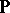),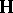(or) and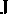are given constitutive equations in terms of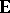,and, respectively. They may also depend on other thermodynamical variables of state, such as temperature and strains (cf. [a8]). Electromagnetic fields have both energy and momentum contents and they contribute to the balance of energy as also to the entropy growth (in the presence of thermodynamically irreversible electromagnetic effects, such as electricity conduction, electric relaxation, magnetic hysteresis, etc.). Maxwell's equations can be viewed as the result of contributions by W. Thomson (Lord Kelvin), H.L.F. Helmholtz, W.E. Weber. J. Henry, and Maxwell, but Maxwell alone is responsible for the introduction of the so-called displacement current (the term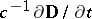), which closed the formulation by giving it a somewhat symmetric form and allowing for the existence of dynamical solutions in terms of electromagnetic waves, experimentally observed by H.R. Hertz in the years 1886–1888. The somewhat blurred introduction of this term made Hertz say that "Maxwell's theory is none other than Maxwell's equations" . As a consequence of (a1)–(a4) one obtains the equation of conservation of electric charges(a6)

Boundary conditions and "jump relations" across surfaces complement (a1)–(a6) in the appropriate mathematical framework, which is that of the theory of generalized functions, or distributions (cf. also Generalized function), as there often exist current sheets and localized point charges (described by means of delta-functions with surface or point-like supports).

It is important to note that the vectors,andare axial, while the vectors,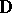andare polar (cf. also Axial vector; Vector analysis). Furthermore, although this does not appear in the above "Euclidean" formulation,andare fundamentally co-vectors, while,andare contra-vectors (one forms). Euclidean physical space does not "see" this difference, but the direct formulation on deformable matter does it in a natural way [a8]. The Maxwell equations given above can also be expressed in a frame co-moving with matter, whether the latter is rigid or deformable. But the passage to another such frame raises the question of "form invariance" of these equations. In a vacuum, Maxwell's equations are trivially Lorentz invariant, that is, they are form-invariant under the Lorentz–Poincaré continuous group of space-time transformations (cf. also Lorentz transformation). In matter, the question is less trivial as all depends on the transformation laws assumed for the material quantitiesand. It is the lack of symmetry for the transformation of these two fields which, together with the constancy of the velocity of light, led to the development of the theory of special relativity by Lorentz, A. Einstein and H. Minkowski (cf. also Relativity theory). However, for engineering purposes, one may "constrain" the material Maxwell equations to be only Galilean invariant. This is obtained in an appropriate Galilean asymptotic limit of small material velocities (see [a8]).

Like other continuum theories (e.g. elasticity), electromagnetism steered the development of numerous fields of pure and applied mathematics and developments in electromagnetism and establishing solutions of many of its problems have enriched mathematics in a typical dialectic movement. The most remarkable fields of mathematics that benefited from electromagnetic studies are:

Potential theory: In electro- and magneto-statics one has the following reductions: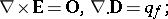(a7)

and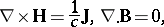(a8)

respectively. In a non-conductor (or isolator),. In a di-electric,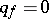and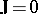. By the first equation in (a7) there exists an electrostatic scalar potentialsuch that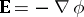. By the second equation in (a8) there exists a vectorial magnetic potentialsuch that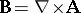. In an isolator, one can also use a magnetostatic scalar potentialsuch that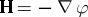. Both scalar and vector potential formulation find their application in finite-element magnetic-field computations.

Vector analysis: The natural setting of Maxwell's equations is vector analysis, for which it was essentially developed through the effort of British scientists in the 19th century (in particular, O. Heaviside).

The theory of quaternions (cf. also Quaternion): This is closely related to vector analysis, but expressed in a more inclusive form. Maxwell himself presents his equations in "quaternionic form" in the first edition (1873) of his celebrated treatise [a1]. The notions of "divergence" and "curl" operations are then united in a single differential operation. Quaternions constitute the generalization of complex numbers to higher dimension and provide an example of a non-commutative algebra.

Gauge theory (cf. also Gauge transformation): Maxwell's electromagnetism is the first successful example of a gauge theory. Equations (a1) and (a2) imply the existence of an electric potentialand a magnetic vector potential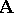such that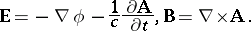(a9)

The Lorentz gauge is such that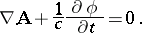(a10)

The theory of continuous groups (cf. also Lie group): The Lorentz–Poincaré invariance of Maxwell's equations was essential in fostering many studies in the theory of continuous groups and in field theories that are solidly based on group-theoretical concepts, such as quantum electrodynamics.

The theory of hyperbolic systems of partial differential equations (cf. also Hyperbolic partial differential equation): The system (a1)–(a4) epitomizes hyperbolic systems of partial differential equations. It was indeed completed by Maxwell, culminating in the prediction of the propagation of waves at a velocity which proved to be none other than that of light, the latter being an upper bound for the propagation velocity of all physical phenomena in classical physics. This boundedness is characteristic of hyperbolicity. Electromagnetic waves of various frequencies and electromagnetic optics were the main two consequences of electromagnetism.

Exterior calculus (cf. also Exterior forms, method of): With the already noticed difference between co-vectors and one-forms, electromagnetism offers the ideal framework for a formulation in terms of the exterior calculus of E. Cartan. Such a formulation of electromagnetism is favoured not only in the fully relativistic background, but also in modern electromagnetic-field computational techniques where facets and edges of the volume discretization play complementary roles. This is similar to Regge calculus.

The combination of electromagnetism and the mechanical equations of motion of charged, electrically polarized and/or magnetized matter provide the object of study of electrodynamics.